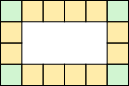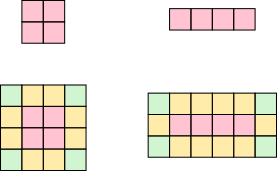#### You may also like### Consecutive Numbers

An investigation involving adding and subtracting sets of consecutive numbers. Lots to find out, lots to explore.### Calendar Capers

Choose any three by three square of dates on a calendar page...### Days and Dates

Investigate how you can work out what day of the week your birthday will be on next year, and the year after...

# Can They Be Equal? Poster

##### Age 11 to 14Challenge Level

Using whole numbers only: 3 by 6 and 4 by 4

Using fractions: Any two numbers $a$ and $b$ where $a\gt2$ and $b = \frac{2a}{a-2}$

Why?

GeometricallyEach square along the edges accounts for one unit of perimeter, except for the four corner squares. They account for two units of perimeter but only one unit of area. This means the perimeter is 4 more than the area. There will have to be 4 squares in the middle that will only be counted for the area and not the perimeter.There are only two ways to make a rectangle using 4 squares.

These make 4 by 4 or 3 by 6 rectangles.

This extends to fractions if the sides of the red rectangle aren't whole numbers, but any two numbers which multiply to 4.

Algebraically
Sides $a$ and $b$

Perimeter: $2a+2b$

Area: $ab$

$2a+2b = ab$

Factorising to get whole number solutions:
$ab - 2a-2b = 0$
$(a-2)(b-2) = \underbrace{ab-2a-2b}_0+4 \therefore (a-2)(b-2)=4$

(This fits with the geometry above because the sides of the red rectangle are $a-2$ and $b-2$)

Whole number solutions: $a-2$ and $b-2$ are $1$ and $3$ or $2$ and $2$
$\therefore a$ and $b$ are $3$ and $6$ or $4$ and $4$

Other solutions: Find any two numbers which multiply to give $4$ and add $2$ to each of them

Rearranging to get infinitely many solutions
\begin{align}ab-2b &= 2a\\ \Rightarrow b(a-2)&=2a\\ \Rightarrow b&=\frac{2a}{a-2}\end{align}

$a$ and $b$ need to be positive $\therefore a\gt2.$ Infinitely many solutions.